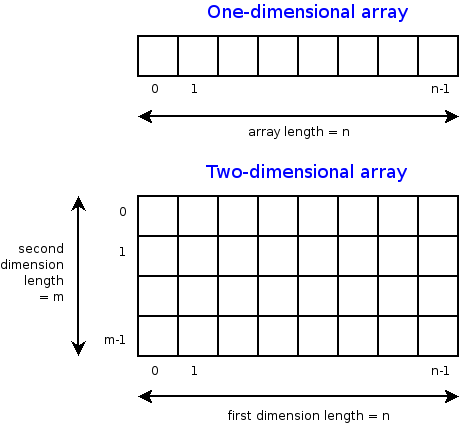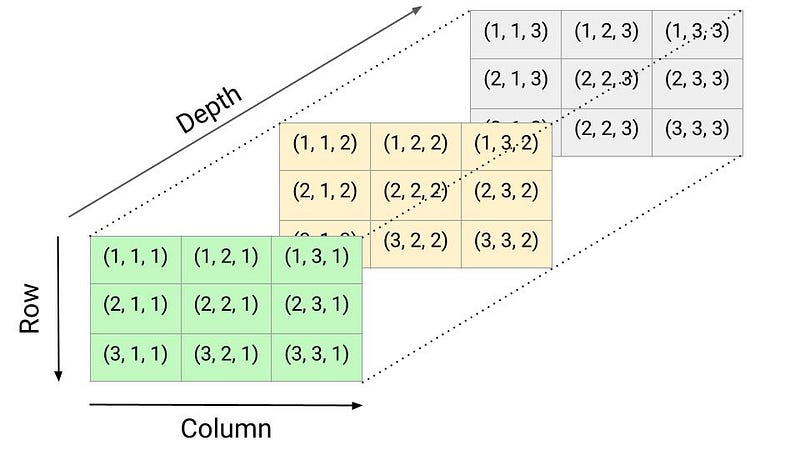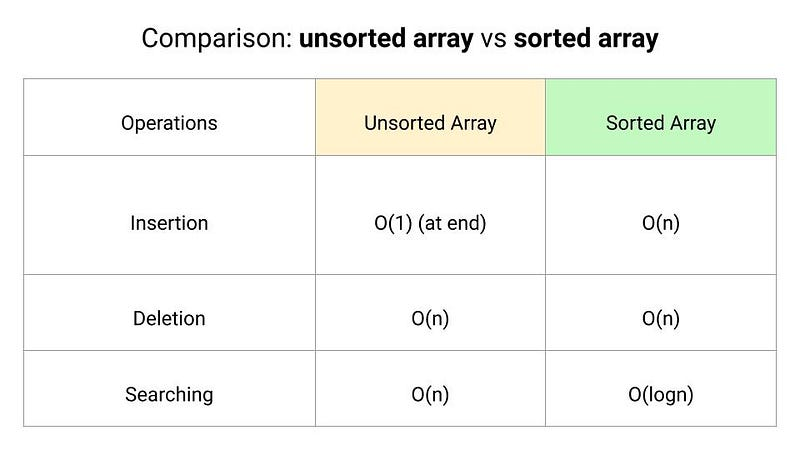# Introduction to Arrays In Data Structures

### Introduction

An array is a contiguous block of memory of the same type of elements where the size is equal to the number of elements in that array, which must be fixed. It is a structure of data such that each element can be efficiently located by its index or memory address.

Using an array, we store multiple items of the same type together. This makes it easier to calculate the position of each element by simply adding an offset to a base value, i.e., the memory location of the first element of the array. The base value is index 0, and the difference between the two indexes is the offset. Remember: The location of the next index depends on the data type we use.

A good analogy of an array is a street full of houses, where each array element is equivalent to a house, and the index is equivalent to the house number. Assuming all the houses are equal in size and numbered sequentially from 1 to n, we can compute the exact position of each house immediately from its address.

Special note on the array

• We can easily calculate the amount of memory that should be allocated to store the array because the size of the array is fixed, and all the elements are of the same type.
• It is easy to compute the starting location of each element in the array, so every element of an array can be accessed in O(1) time.
• 0-based array indexing is a way of numbering the elements in an array such that the first element is present at the 0th index, whereas a 1-based array indexing has its first element present at index 1. 0-based indexing is a very common way to number items in a sequence in today’s modern mathematical notation. Even most programming languages follow the standard of 0-based indexing.

### Types of arrays

One-dimensional array or 1-D array

In a one-dimensional array, the elements are stored in contiguous memory locations where each element is accessed using a single index value. It is a linear data structure storing all the elements in a sequence.

The elements are stored in a continuation memory location, so the variable declared as an array is a pointer to the address of the first element of the array. This address is called the base address. The address of any other element can be calculated with the following formula:

Memory Address (A[i])= Base Address (A) + Word Length * ( i - First Index)

• Memory Address: memory location of the ith element of the array.
• Word Length: number of bytes required to store one element depending upon its data type, like character, integer, float, double, etc.
• First Index: Index of the first element of the array.

Two-dimensional array or 2-D array

A two-dimensional array is a tabular representation where elements are stored in rows and columns. It is a collection of m X n elements which has m rows and n columns. To access any element in a 2-D array, two pieces of information are required to define an element's position in a specific row and column: 1) the first index of row 2) Column index.

We primarily represent a 2-D array in a row-major representation where the storage of array elements takes place row-wise. All elements of the first row of the array are first stored in sequence, followed by the second row and then third, fourth, and so on. We can find the address of any element located at the ith row and the jth column using the following formula:

Memory Address(A[i, j])= Base Address(A)+ Word Length * (n * (i-1) + (j-1))Three-dimensional array or 3-D array

A three-dimensional array is an extension to the two-dimensional array with the addition of depth. It can be seen as a cube with rows, columns, and depth as the third dimension. To access any element in a three-dimensional array, three pieces of information are required for the position of an element in a specific row, column, and depth: 1) The first index of the third dimension i.e. depth 2) Row index 3) Column index### Some popular array operations

Now we know the basic idea behind an array, let's look at the various operations that can be performed on arrays.

• Traverse: Accesselements in the array one by one.
• Insertion: Adds an element at the given index.
• Deletion: Deletes an element at the given index.
• Search: Searches an element in the array using the given index or the value.
• Update: Updates an element at the given index.
• Sorting: Arrange elements in increasing or decreasing order.
• Merging: Merge two sorted arrays into a larger sorted array
• Order statistics: Find the kth smallest/largest element in an array

### Search, insert and delete in an unsorted array

Search Operation

In an unsorted array, we search elements using the linear search.

``````int linearSearch(int A[], int n, int key)
{
for (int i = 0; i < n; i = i + 1)
if (A[i] == key)
return i
return -1
}
``````

The worst-case time complexity of the linear search is O(n).

Insert Operation

In an unsorted array, there is no order associated with the data elements. So we can directly insert elements at the end of the array. Suppose the size of the array is n and the index of the end element is endIndex.

• if(endIndex < n), then there is some space left in the array and we can insert the key at the end i.e. at index endIndex + 1. As an output of this process, we also return the new index of the end element which is endIndex + 1.
• if(endIndex >= n), then the array is full and it is not possible to insert any element further. We return the endIndex value as an output.
``````int insert(int A[], int endIndex, int key, int n)
{
if(endIndex >= n)
return endIndex
A[endIndex + 1] = key
return endIndex + 1
}
``````

The time complexity of the insert operation is O(1).

Delete Operation

• We first search for the key to be deleted using the linear search. Suppose linear search returns the index of the key in the array, which we store in the variable currIndex.
• If (currIndex == -1), then the element to be deleted is not present in the array. We return the value of the last index i.e. endIndex.
• Otherwise, the key is present in the array. We delete the key by shifting all elements to the one left from index currIndex + 1 to endIndex.
``````int delete(int A[], int endIndex, int key)
{
int currIndex = linearSearch(A, endIndex, key)
if (currIndex == - 1)
return endIndex
for (int i = currIndex; i < endIndex; i = i + 1)
A[i] = A[i + 1]
return endIndex - 1
}
``````

Time complexity = Time complexity of the linear search + Time complexity of shifting elements to the left = O(n) + O(n) = O(n)

### Search, insert and delete in a sorted array

Search Operation
In a sorted array, we search elements using the binary search.

``````int binarySearch(int A[], int l, int r, int key)
{
if (l > l)
return -1
int mid = l + (r - l)/2
if (key == A[mid])
return mid

if (key > A[mid])
return binarySearch(A, mid + 1, r, key)
return binarySearch(A, l, mid - 1, key)
}
``````

The worst-case time complexity of the binary search is O(logn).

Insert Operation

In a sorted array, a search operation is performed to find the position of the given element by using binary search, and then the insert operation is performed followed by shifting elements to one right.

``````int insertSorted(int A[], int endIndex, int key, int n)
{
if(endIndex >= n)
return endIndex

for(int i = endIndex - 1; i >= 0 && A[i] > key; i = i - 1)
A[i + 1] = A[i]

A[i + 1] = key
return endIndex + 1
}
``````

Time complexity = Time complexity of the binary search + Time complexity of shifting elements to the right = O(logn) + O(n) = O(n)

Note: In an unsorted array, insertion is faster as compared to a sorted array because we don’t have to care about the position at which the element is to be placed.

Delete Operation

In the delete operation, the element to be deleted is searched using binary search, and then the delete operation is performed followed by shifting the elements to the one left.

``````int deleteSorted(int A[], int endIndex, int key)
{
int currIndex = binarySearch(A, 0, endIndex, key)

if (currIndex == -1)
return endIndex

for (int i = currIndex; i < endIndex; i = i + 1)
A[i] = A[i + 1]

return endIndex - 1
}
``````

Time complexity = Time complexity of the binary search + Time complexity of shifting elements to the left = O(logn) + O(n) = O(n)Constant time access: The index of each element in an array maps directly to a particular memory address. So we can access the data element instantly if we know the index of a data element in an array.

Optimal use of memory: Arrays consist purely of data, so no space is wasted with links or other formatting information.

Memory locality: Most programming problems require iterating through all the elements of a data structure. So arrays are good for this because successive data elements are present in sequential order, exhibiting excellent memory locality. This property helps us to implement high-speed cache memory on modern computer architectures.

The main drawbacks of using arrays are their restrictions. Arrays cannot be used to store elements of different types (or sizes), and the size of an array cannot be changed dynamically. In other words, we cannot adjust the size of the array in the middle of a program’s execution. Our code will fail soon as we try to add the (n+1)st data element if we only allocate space for n data elements. We can compensate for this by allocating large arrays, but this can also waste a lot of space. To solve the above problem, we can use the idea of the dynamic array where we can increase the array size dynamically as we need them.

### Array-based data structures

• Array implementation of stack
• Array implementation of queue
• Array implementation of double-ended queue
• Array implementation of binary heap
• Array implementation of priority queue
• Array implementation of Hash tables (open addressing method)
• Graph implementation using the adjacency matrix

### Critical ideas to think!

• Which Indexing is more effcient: 0-based indexing or 1-based indexing?
• How does a dynamic array solve fixed array size problems?
• What type of data structure do we use to store multiple types of items in a continuous memory location?
• How can we find the length of an array, given only the name of the array?
• What is the use/application of 3D arrays?
• How can we insert or delete a new element at the beginning of an unsorted or sorted array?

### Popular array-based problem-solving approaches

• Building partial solutions using a single loop
• Two-pointers approach
• Sliding window approach
• Divide and conquer approach
• Decrease and conquer
• Using the idea of binary search
• Using sorting and loop
• Using extra space and loop
• Bit manipulation approach

### Applications of array

• Arranging a particular type of data in a sequential arrangement: storing contacts on our phone, storing speech signals in speech processing, etc.
• Implementing of Stack and Queue, Adjacency matrix representation of Graphs, Implementing hash tables and heaps.

### Suggested array-based problems to practice

Enjoy learning, Enjoy Algorithms!

Get well-designed application and interview centirc content on ds-algorithms, machine learning, system design and oops. Content will be delivered weekly.